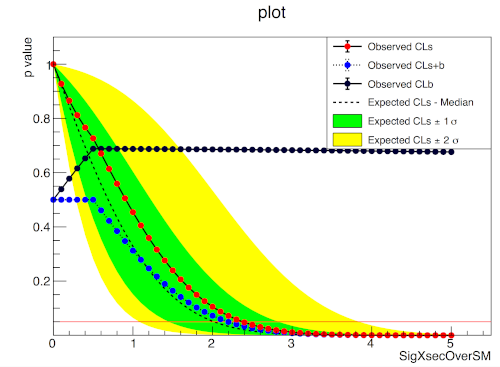Fetching contributors…Cannot retrieve contributors at this time
114 lines (83 sloc) 5.32 KB

# pure-python fitting/limit-setting/interval estimation HistFactory-styleThe HistFactory p.d.f. template [CERN-OPEN-2012-016] is per-se independent of its implementation in ROOT and sometimes, it's useful to be able to run statistical analysis outside of ROOT, RooFit, RooStats framework.

This repo is a pure-python implementation of that statistical model for multi-bin histogram-based analysis and its interval estimation is based on the asymptotic formulas of "Asymptotic formulae for likelihood-based tests of new physics" [arxiv:1007.1727]. The aim is also to support modern computational graph libraries such as PyTorch and TensorFlow in order to make use of features such as autodifferentiation and GPU acceleration.

## Hello World

```>>> import pyhf
>>> pdf = pyhf.simplemodels.hepdata_like(signal_data=[12.0, 11.0], bkg_data=[50.0, 52.0], bkg_uncerts=[3.0, 7.0])
>>> CLs_obs, CLs_exp = pyhf.utils.hypotest(1.0, [51, 48] + pdf.config.auxdata, pdf, return_expected=True)
>>> print('Observed: {}, Expected: {}'.format(CLs_obs, CLs_exp))
Observed: [0.05290116], Expected: [0.06445521]
```

## What does it support

Implemented variations:

• HistoSys
• OverallSys
• ShapeSys
• NormFactor
• Multiple Channels
• Import from XML + ROOT via uproot
• ShapeFactor
• StatError
• Lumi Uncertainty

Computational Backends:

• NumPy
• PyTorch
• TensorFlow

Available Optimizers

NumPy Tensorflow PyTorch
SLSQP (`scipy.optimize`) Newton's Method (autodiff) Newton's Method (autodiff)
MINUIT (`iminuit`) . .

## Todo

• StatConfig
• Non-asymptotic calculators

results obtained from this package are validated against output computed from HistFactory workspaces

## A one bin example

``````nobs = 55, b = 50, db = 7, nom_sig = 10.
``````## A two bin example

``````bin 1: nobs = 100, b = 100, db = 15., nom_sig = 30.
bin 2: nobs = 145, b = 150, db = 20., nom_sig = 45.
``````## Installation

To install `pyhf` from PyPI with the NumPy backend run

``````pip install pyhf
``````

and to install `pyhf` with additional backends run

``````pip install pyhf[tensorflow,torch]
``````

or a subset of the options.

To uninstall run

`pip uninstall pyhf`

## Authors

Please check the contribution statistics for a list of contributors

You can’t perform that action at this time.